# Basic Electronics - JFET

The JFET is abbreviated as Junction Field Effect Transistor. JFET is just like a normal FET. The types of JFET are n-channel FET and P-channel FET. A p-type material is added to the n-type substrate in n-channel FET, whereas an n-type material is added to the ptype substrate in p-channel FET. Hence it is enough to discuss one type of FET to understand both.

## N-Channel FET

The N-channel FET is the mostly used Field Effect Transistor. For the fabrication of Nchannel FET, a narrow bar of N-type semiconductor is taken on which P-type material is formed by diffusion on the opposite sides. These two sides are joined to draw a single connection for gate terminal. This can be understood from the following figure.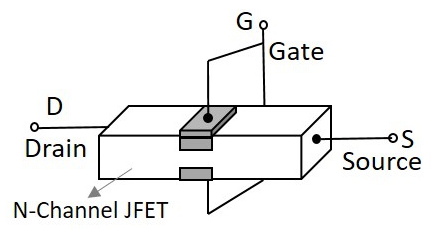These two gate depositions (p-type materials) form two PN diodes. The area between gates is called as a channel. The majority carriers pass through this channel. Hence the cross sectional form of the FET is understood as the following figure.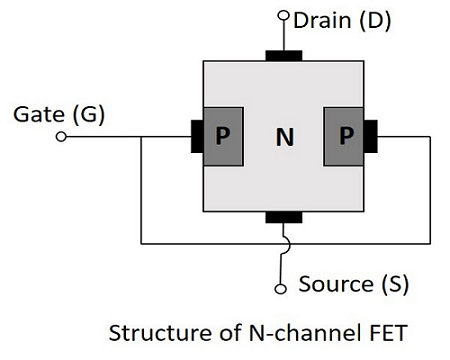Ohmic contacts are made at the two ends of the n-type semiconductor bar, which form the source and the drain. The source and the drain terminals may be interchanged.

## Operation of N-channel FET

Before going into the operation of the FET one should understand how the depletion layers are formed. For this, let us suppose that the voltage at gate terminal say VGG is reverse biased while the voltage at drain terminal say VDD is not applied. Let this be the case 1.

• In case 1, When VGG is reverse biased and VDD is not applied, the depletion regions between P and N layers tend to expand. This happens as the negative voltage applied, attracts the holes from the p-type layer towards the gate terminal.

• In case 2, When VDD is applied (positive terminal to drain and negative terminal to source) and VGG is not applied, the electrons flow from source to drain which constitute the drain current ID.

Let us now consider the following figure, to understand what happens when both the supplies are given.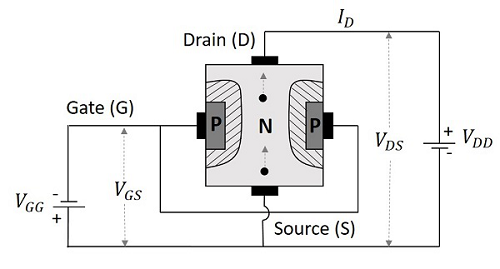The supply at gate terminal makes the depletion layer grow and the voltage at drain terminal allows the drain current from source to drain terminal. Suppose the point at source terminal is B and the point at drain terminal is A, then the resistance of the channel will be such that the voltage drop at the terminal A is greater than the voltage drop at the terminal B. Which means,

VA>VB

Hence the voltage drop is being progressive through the length of the channel. So, the reverse biasing effect is stronger at drain terminal than at the source terminal. This is why the depletion layer tends to penetrate more into the channel at point A than at point B, when both VGG and VDD are applied. The following figure explains this.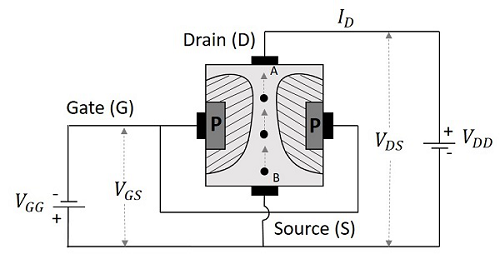Now that we have understood the behavior of FET, let us go through the real operation of FET.

### Depletion Mode of Operation

As the width of depletion layer plays an important role in the operation of FET, the name depletion mode of operation implies. We have another mode called enhancement mode of operation, which will be discussed in the operation of MOSFETs. But JFETs have only depletion mode of operation.

Let us consider that there is no potential applied between gate and source terminals and a potential VDD is applied between drain and source. Now, a current ID flows from drain to source terminal, at its maximum as the channel width is more. Let the voltage applied between gate and source terminal VGG is reverse biased. This increases the depletion width, as discussed above. As the layers grow, the cross-section of the channel decreases and hence the drain current ID also decreases.

When this drain current is further increased, a stage occurs where both the depletion layers touch each other, and prevent the current ID flow. This is clearly shown in the following figure.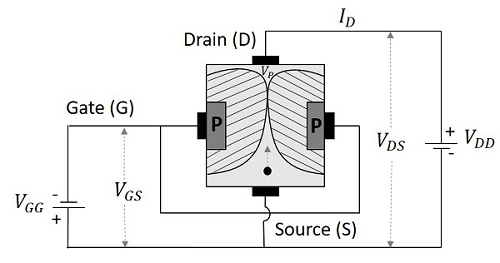The voltage at which both these depletion layers literally “touch” is called as “Pinch off voltage”. It is indicated as VP. The drain current is literally nil at this point. Hence the drain current is a function of reverse bias voltage at gate.

Since gate voltage controls the drain current, FET is called as the voltage controlled device. This is more clearly understood from the drain characteristics curve.

## Drain Characteristics of JFET

Let us try to summarize the function of FET through which we can obtain the characteristic curve for drain of FET. The circuit of FET to obtain these characteristics is given below.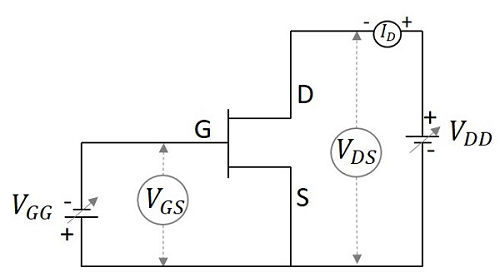When the voltage between gate and source VGS is zero, or they are shorted, the current ID from source to drain is also nil as there is no VDS applied. As the voltage between drain and source VDS is increased, the current flow ID from source to drain increases. This increase in current is linear up to a certain point A, known as Knee Voltage.

The gate terminals will be under reverse biased condition and as ID increases, the depletion regions tend to constrict. This constriction is unequal in length making these regions come closer at drain and farther at drain, which leads to pinch off voltage. The pinch off voltage is defined as the minimum drain to source voltage where the drain current approaches a constant value (saturation value). The point at which this pinch off voltage occurs is called as Pinch off point, denoted as B.

As VDS is further increased, the channel resistance also increases in such a way that ID practically remains constant. The region BC is known as saturation region or amplifier region. All these along with the points A, B and C are plotted in the graph below.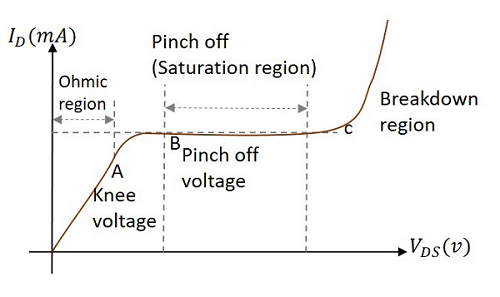The drain characteristics are plotted for drain current ID against drain source voltage VDS for different values of gate source voltage VGS. The overall drain characteristics for such various input voltages is as given under.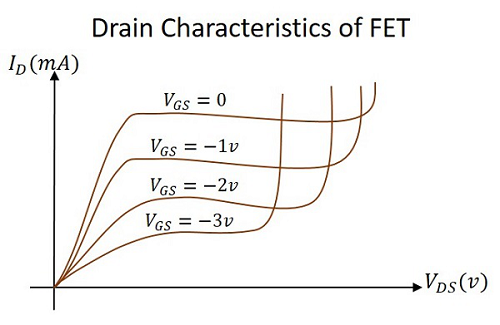As the negative gate voltage controls the drain current, FET is called as a Voltage controlled device. The drain characteristics indicate the performance of a FET. The drain characteristics plotted above are used to obtain the values of Drain resistance, Transconductance and Amplification Factor.